# avasms

avasms computes avas using a series of alternative options

## Syntax

• BestSol=avasms(y,X)example
• BestSol=avasms(y,X,Name,Value)example
• [BestSol,corMatrix]=avasms(___)example

## Description

This function applies avas with a series of options and produces the augmented star plot.

Below we list the five options giving an informative text description followed by the optional input arguments. We also give in parenthesis, the abbreviations used in the augmented star plot, where these are different.

- Robustness (rob).

- Remove effect of order of explanatory variables by regression (scail).

- Trapezoidal or rectangular rule for numerical integration in function ctsub (trapezoid).

- Ordering inclusion of the variables in the backfitting algorithm using $R^2$ values (orderR2).

- Initial robust transformation of the response (tyinitial).

We reduce the number of analyses for investigation by removing all those for which the residuals fail the Durbin-Watson and Jarque-Bera tests, at the 10 per cent level (two-sided for Durbin-Watson). Unlike the Durbin-Watson test, the Jarque-Bera test uses a combination of estimated skewness and kurtosis to test for distributional shape of the residuals.

Note that this threshold of 10 per cent can be changed using optional input argument critBestSol.

We order the solutions by the Durbin-Watson significance level multiplied by the value of $R^2$ and by the number of units not declared as outliers. The rays in individual plots are of equal length for those features used in an analysis. All rays are in identical places in each plot. Information in the plot is augmented by making the length of the rays for each analysis reflect the properties of the analysis; they are proportional to $p_{DW}$, the significance level of the Durbin-Watson test. Note that the ordering in which the solutions are displayed in the plot can be changed using optional input argument SolutionOrdering.

The five options start on the right and wind counterclockwise in steps of 72 degrees around the circle. The ordering in which the five options are displayed in the plot depends on the frequency of presence among the set of the admissible solutions. For example, if robustness is the one who has the highest frequency, its spoke is shown on the right (plotted to the East). The second most present option is shown on the top right...

and the least present option is shown on the bottom right.

 BestSol =avasms(y, X) Example 2 in RAC (2022).

 BestSol =avasms(y, X, Name, Value) Wang and Murphy data.

 [BestSol, corMatrix] =avasms(___) Example of the use of avasms using the Fish data.

## Examples

expand all

###Example 2 in RAC (2022).

There are four explanatory variables and 151 observations with $x_1$ equally spaced from 0:0.1:15. The linear model is: $z = \text{sin}(x) + \sum_{j=2}^4 (j-1)x_j + 0.5\mathcal{U}(-0.5,0.5),$ where $x_{ij} (j=2, \ldots, 4),$ are independently $\mathcal{N}(0,1).$ Eight outliers of value one replace the values of $z$ at $x = 8.9, 9.9, 10.4, 10.5 ... 10.9$. The response $y = \exp z$. There are thus four explanatory variables, only one of which requires transformation, as does the response to log$y$.

rng(100)
x1 = (0:0.1:15)';
n=length(x1);
X3=randn(n,3);
Xb=X3*(1:3)';
z = sin(x1) + 0.5*(rand(size(x1))-0.5)+Xb;
z([90,100,105:110]) = 1;
X=[x1 X3];
y=exp(z);
% Automatic model selection
[BestSol,corMatrix]=avasms(y,X);
No model found with pvalDW>0.1 and pvalJB>0.1
Setting pvalDW=0.05 and pvalJB=0.05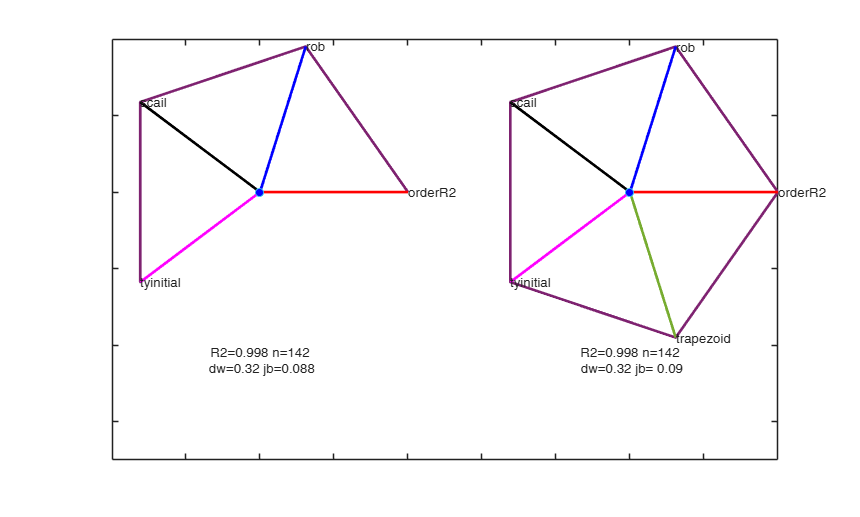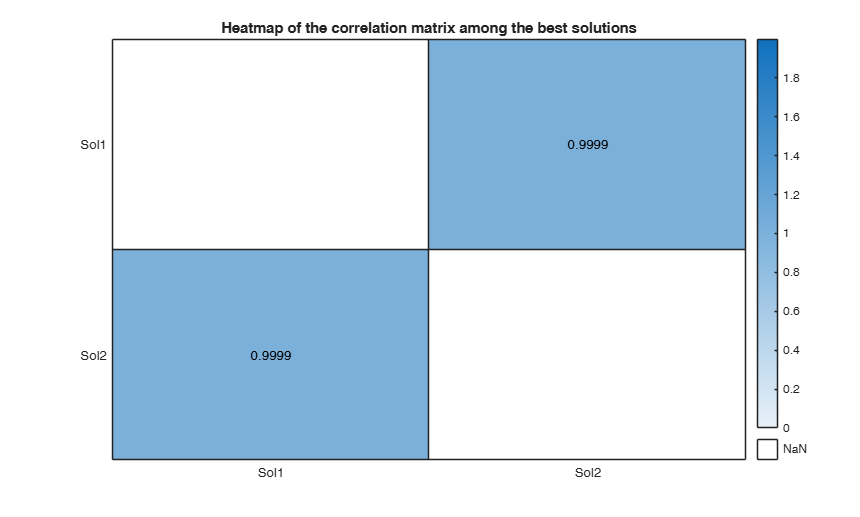###Wang and Murphy data.

rng('default')
seed=100;
negstate=-30;
n=200;
X1 = mtR(n,0,seed)*2-1;
X2 = mtR(n,0,negstate)*2-1;
X3 = mtR(n,0,negstate)*2-1;
X4 = mtR(n,0,negstate)*2-1;
res=mtR(n,1,negstate);
% Generate y
y = log(4 + sin(3*X1) + abs(X2) + X3.^2 + X4 + .1*res );
X = [X1 X2 X3 X4];
y([121 80 34 188 137 110 79 86 1])=1.9+randn(9,1)*0.01;
[BestSol,corMatrix]=avasms(y,X);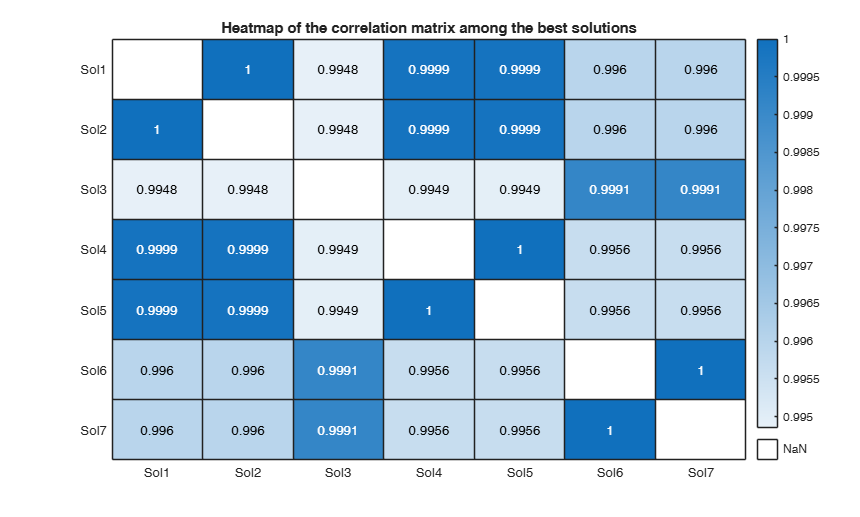###Example of the use of avasms using the Fish data.

load("fish.mat");
Y=fish;
% pike is removed. We use just the first 3 explanatory variables.
sel=categorical(Y{:,1})~='Pike';
y=Y{sel,2};
X=Y{sel,3:5};
[BestSol,corMatrix]=avasms(y,X,'l',3*ones(size(X,2),1));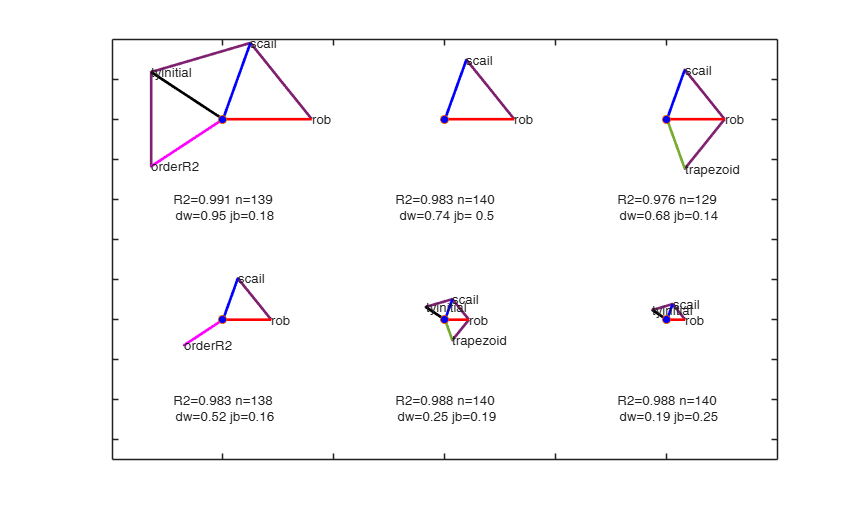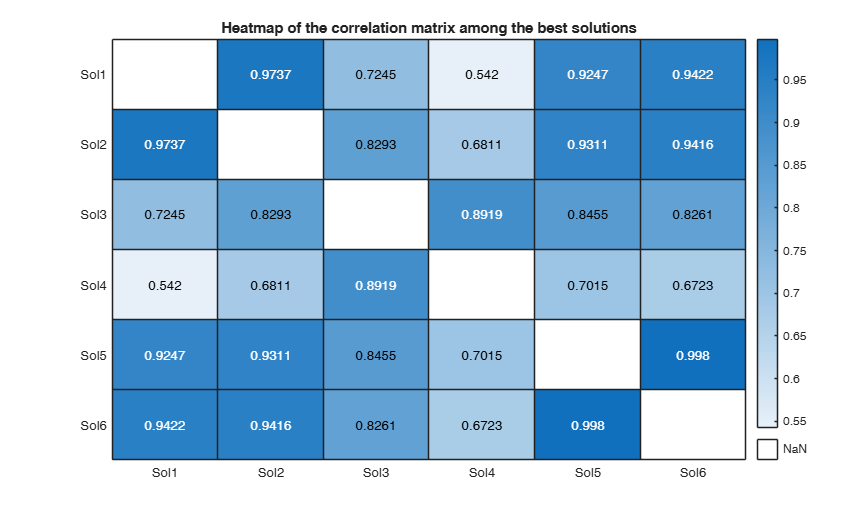## Related Examples

expand all

### Example of use of option critBestSol with marketing data.

load("Marketing_Data.mat")
Y=Marketing_Data;
y=Y{:,4};
X=Y{:,1:3};
% In this case the admissible solutions are defined as those which have a
% p-value of DW test and a p-value of JB test greater than 0.001
critBestSol=0.001;
% Note that if a different threshold for the two p-values is needed we have
% to define critBestSol as a struct as follows
%  critBestSol=struct;
%  critBestSol.pvalDW=0.0001;
%  critBestSol.pvalJB=0.001;
% In this example it makes sense to force a monotonic transformation for the
% 3 explanatory variables
out=avasms(y,X,'l',ones(3,1),'critBestSol',critBestSol);

## Input Arguments

### y — Response variable. Vector.

Response variable, specified as a vector of length n, where n is the number of observations. Each entry in y is the response for the corresponding row of X.

Missing values (NaN's) and infinite values (Inf's) are allowed, since observations (rows) with missing or infinite values will automatically be excluded from the computations.

Data Types: single| double

### X — Predictor variables. Matrix.

Matrix of explanatory variables (also called 'regressors') of dimension n x (p-1) where p denotes the number of explanatory variables including the intercept.

Rows of X represent observations, and columns represent variables. By default, there is a constant term in the model, unless you explicitly remove it using input option intercept, so do not include a column of 1s in X. Missing values (NaN's) and infinite values (Inf's) are allowed, since observations (rows) with missing or infinite values will automatically be excluded from the computations.

Data Types: single| double

### Name-Value Pair Arguments

Specify optional comma-separated pairs of Name,Value arguments. Name is the argument name and Value is the corresponding value. Name must appear inside single quotes (' '). You can specify several name and value pair arguments in any order as  Name1,Value1,...,NameN,ValueN.

Example:  'critBestSol',0.001 , 'delrsq',0.001 , 'l',[3 3 1] , 'maxit',30 , 'maxBestSol',5 , 'nterm',5 , solOrdering,["R2" "pvalJB"] , 'w',1:n , 'plots',true , 'showBars',true 

### critBestSol —criterion to define the admissible solutions to retain.scalar | struct.

The default value of critBestSol is 0.10, that is solutions are retained if the associated residuals pass the Durbin-Watson and Jarque-Bera tests, at the 10 per cent level. For example if critBestSol is 0.01, solutions are retained if the associated residuals pass the Durbin-Watson and Jarque-Bera tests, at the 1 per cent level. If critBestSol is a scalar the p-value threshold is the same both for Durbin-Watson and Jarque-Bera tests. On the other hand, if critBestSol is a struct it is possible to use a different p-value threshold for each test. If critBestSol is a struct it may contain the following fields:

Value Description
pvalDW

threshold for the p-value of Durbin Watson test (if this field is not present default is critBestSol.pvalDW=0.10).

pvalJB

threshold for the p-value of Jarque-Bera test (if this field is not present default is critBestSol.pvalJB=0.10).

Example:  'critBestSol',0.001 

Data Types: double or struct.

### delrsq —termination threshold.scalar.

Iteration (in the outer loop) stops when rsq changes less than delrsq in nterm. The default value of delrsq is 0.01.

Example:  'delrsq',0.001 

Data Types: double

### l —type of transformation.vector.

Vector of length p which specifies the type of transformation for the explanatory variables.

l(j)=1 => j-th variable assumes orderable values and any transformation (also non monotone) is allowed.

l(j)=2 => j-th variable assumes circular (periodic) values in the range (0.0,1.0) with period 1.0.

l(j)=3 => j-th variable transformation is to be monotone.

l(j)=4 => j-th variable transformation is to be linear.

l(j)=5 => j-th variable assumes categorical (unorderable) values.

j =1, 2,..., p.

The default value of l is a vector of ones of length p, that is the procedure assumes that all the explanatory variables have orderable values (and therefore can be transformed non monotonically).

Note that in avas procedure the response is always transformed (in a monothonic way).

Example:  'l',[3 3 1] 

Data Types: double

### maxit —maximum number of iterations for the outer loop.scalar.

The default maximum number of iterations before exiting the outer loop is 20.

Example:  'maxit',30 

Data Types: double

### maxBestSol —criterion to define the maximum number of admissible solutions to show in the augmented star plot.positive integer scalar.

The default value of maxBestSol is 8 that is a maximum of 8 solutions is shown in the plot.

Note that this is the upper bound among the set of the admissible solutions. If the number of admissible solutions is smaller than maxBestSol then this optional input argument is ignored.

Example:  'maxBestSol',5 

Data Types: double

### nterm —minimum number of consecutive iterations below the threshold to terminate the outer loop.positive scalar.

This value specifies how many consecutive iterations below the threshold it is necessary to have to declare convergence in the outer loop. The default value of nterm is 3.

Example:  'nterm',5 

Data Types: double

### solOrdering —criterion to order the solutions in the augmented star plot.cell array of characters | array of strings.

The elements of the cell are the names of columns 6-9 of the table in output argument BestSol. More precisely, these names are "R2" "pvalDW" "pvalJB" "nused". For example, if solOrdering=["R2" "pvalDW"] or solOrdering={'R2' 'pvalDW'}, the ordering of the solutions is based on the product between the values of R2 and that of the p-value of DW test. If this optional input argument is not specified or it is empty, the default is to use solOrdering=["R2" "pvalDW" "nused"], that is the product of the p-value of Durbin Watson test, the value of R2 and the number of units which have not been declared as outliers.

Example:  solOrdering,["R2" "pvalJB"] 

Data Types: Cell array of characters or array of strings

### w —weights for the observations.vector.

Row or column vector of length n containing the weights associated to each observations. If w is not specified we assume $w=1$ for $i=1, 2, \ldots, n$.

Example:  'w',1:n 

Data Types: double

### plots —plots on the screen.boolean.

If plots is true it is possible to visualize on the screen the augmented star plot and the heatmap associated to the correlation among the admissible solutions. The plot which contains the augmented start plot is tagged pl_augstar, while the plot which contains the heatmap is tagged pl_heatmap. The default value of plots is false, that is no plot is shown on the screen.

Example:  'plots',true 

Data Types: Logical

### showBars —show bars instead of text.boolean.

If showBars is true the values of R2, fraction of units used, pvalue of DW test and pval of normality test are shown with bars below each star, else (default) these values are shown using a textbox.

Example:  'showBars',true 

Data Types: logical

## Output Arguments

### BestSol —Best solutions. table

A table containing the details of the admissible solutions which have been found. We define a solution as admissible if the residuals pass the Durbin-Watson and Jarque-Bera tests, at the 10 per cent level.

If no solution is found, than a 5 per cent threshold for both tests is used.

Note that in input option critBestSol it is possible to set up different thresholds to define the admissible solutions.

The rows of BestSol are ordered in a non increasing way using the p-value of the Durbin Watson test (rescaled by the value of $R^22$ and the number of units not declared as outliers).

Colums 1-5 contain boolean information about the usage of options: orderR2, scail, trapezoid, rob, tyinitial. These 5 columns are ordered in non decreasing way depending on the frequency in which a particular option has been used in the set of admissiable solutions.

For example, if option orderR2 is the only one which is always used in the set of admissible solutions, then column 1 is associated to orderR2.

6th column contains the value of R2 (column name R2).

7th column contains the p-value of Durbin Watson test (column name pvalDW).

8th column contains the p-value of Jarque Bera test (column name pvalJB).

9th column contains the number of units which have not been declared as outliers (column name nused).

10th column is a cell which contains the residuals for the associated solution.

11th column contains the struct out which is the output of the call to avas.

12th column contains the numbers obtained by the product of p-value of Durbin Watson test, the values of $R^2$ and the number of units which have not been declared as outliers. The values of this column depend on the optional input argument solOrdering.

### corMatrix —Correlation matrix.  Correlation matrix among the residuals associated to the admissible solutions which have been found

The size of CorMatrix is size(BestSol,1)-times-size(BestSol,1).

Routine avas, (additivity and variance stabilization) provides non-parametric alternatives to the widely used Box-Cox parametric transformation of the response and the Box-Tidwell family of power transformations of explanatory variables. In routine avas it is possible:

 To use option rob in order to replace standard OLS regression with robust regression in all steps of the iterative procedure. More precisely using robust regression we identify a subset $S_m$ of clean observations to use in the backfitting algorithm to calculate the transformations $f(X)$ at each iteration, the $n-m$ outliers being ignored. We also use the subset in function ctsub in the calculation of the numerical variance stabilising transformation. Since different response transformations can indicate different observations as outliers, the identification of outliers occurs repeatedly during our robust algorithm, once per iteration.

 To use option scail in order to linearly transform the explanatory variables in order to let each column of matrix X have the same weight when predicting g(y).

 To use option orderR2 to completely eliminate dependence on the order of the variables. That is, in each iteration of the backfitting algorithm we impose an ordering which is based on the variable which produces the highest increment of $R^2$.

 To use option tyinitial which calculates a robust parametric transformation of the response before starting the main backfitting loop.

 To use option trapeziod in order to specify how to compute the integral to obtain transformed values for the units which fall outside the range of integration determined by the units not declared as outliers.

The purpose of this routine is to apply avas with and without the five options listed above. There are therefore 32 combinations of options that could be chosen. It is not obvious that all will be necessary when analysing all sets of data. This routine returns the list of options which produced the best fit and provides the necessary ingredients for a graphical method, the augmented star plot, to indicate which options are important in a particular data analysis.

Riani M., Atkinson A.C. and Corbellini A. (2022), Robust Transformations for Multiple Regression via Additivity and Variance Stabilization, submitted.

Tibshirani R. (1987), Estimating optimal transformations for regression, "Journal of the American Statistical Association", Vol. 83, 394-405.

Wang D. and Murphy M. (2005), Identifying nonlinear relationships regression using the ACE algorithm, "Journal of Applied Statistics", Vol. 32, pp. 243-258.